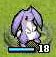# 星之一角

What have you found for these years?

## 2009-12-09

`trait Monad[+a]{  def >>=[b, c <: Monad[b]](f: a => c): c}abstract class Maybe[+a] extends Monad[a]{  override  def >>=[b, c <: Monad[b]](f: a => c): c = this match{    // case Void => Void    case Just(a) => f(a)  }}case object Void extends Maybe[Nothing]case class Just[+a](aa: a) extends Maybe[a]println(Just(1).>>=[Int, Just[Int]]((x: Int) => Just(x * 2)))`

==
* 另一方面，今天狀況很不好，還有待調整 ><
* computer science 不知不覺就聊得很兇... 結果進度全亂了 囧>
* bah, 我快累昏了，要睡要睡要睡，啥屁話以後再說

#### 4 retries: